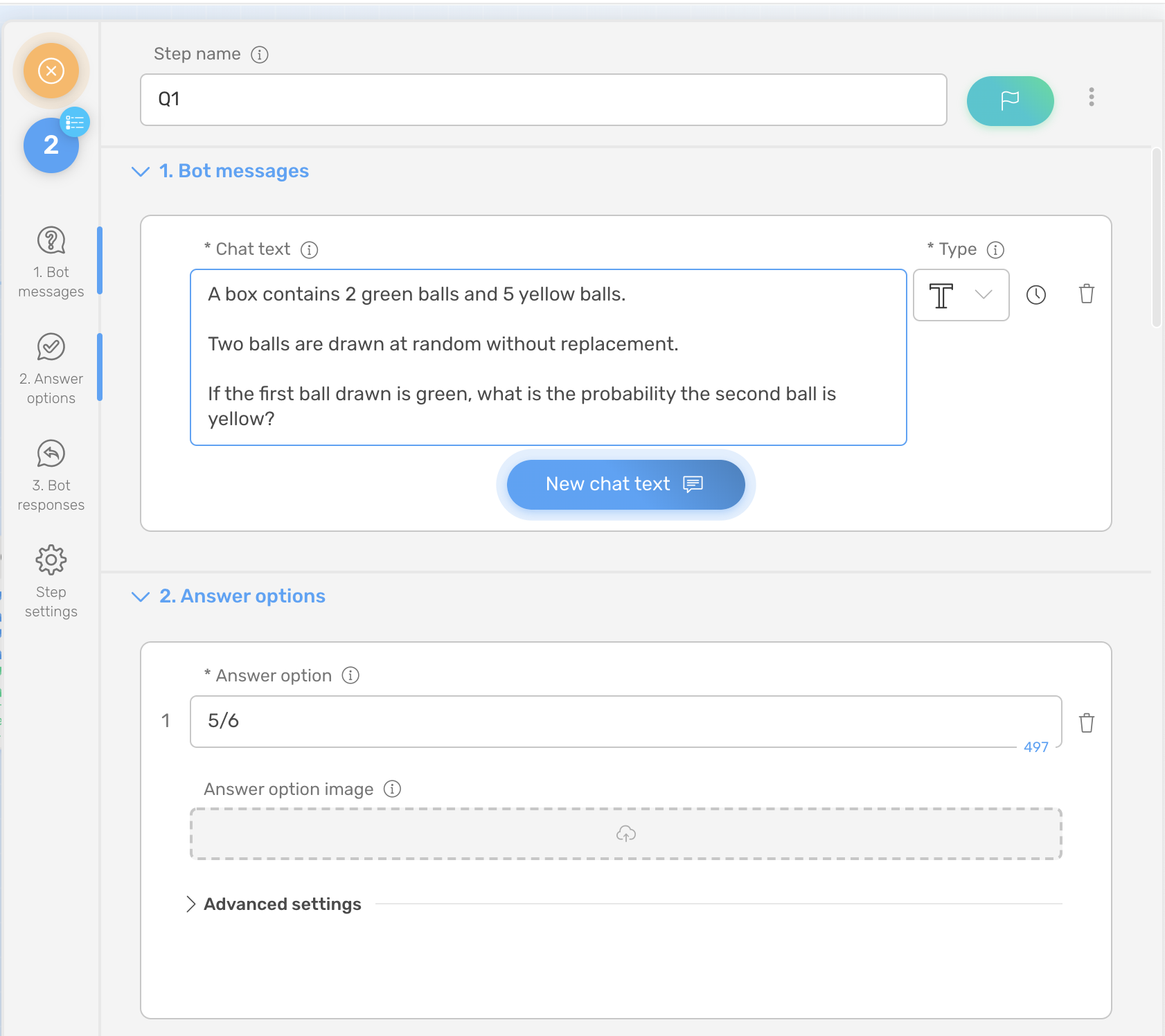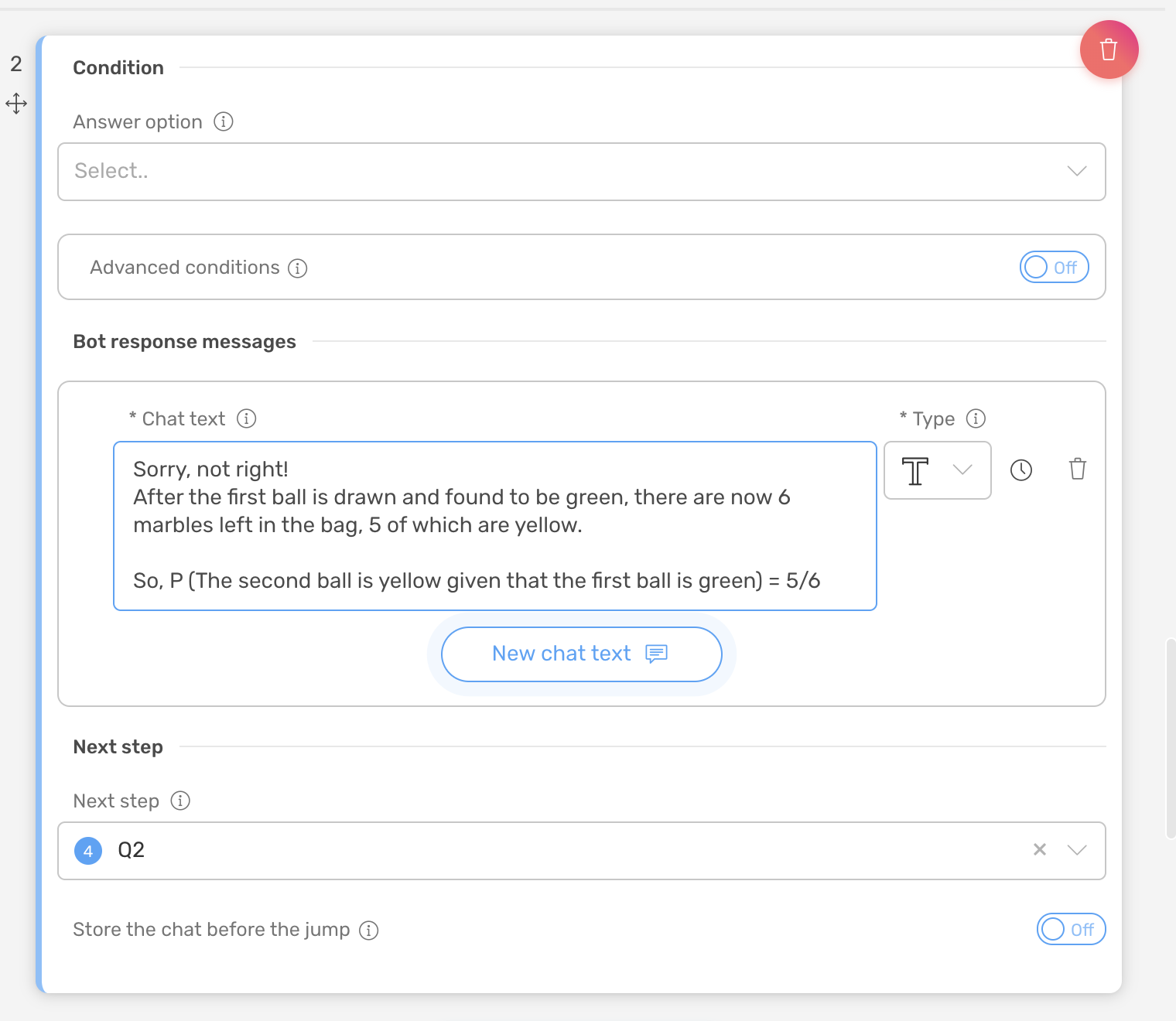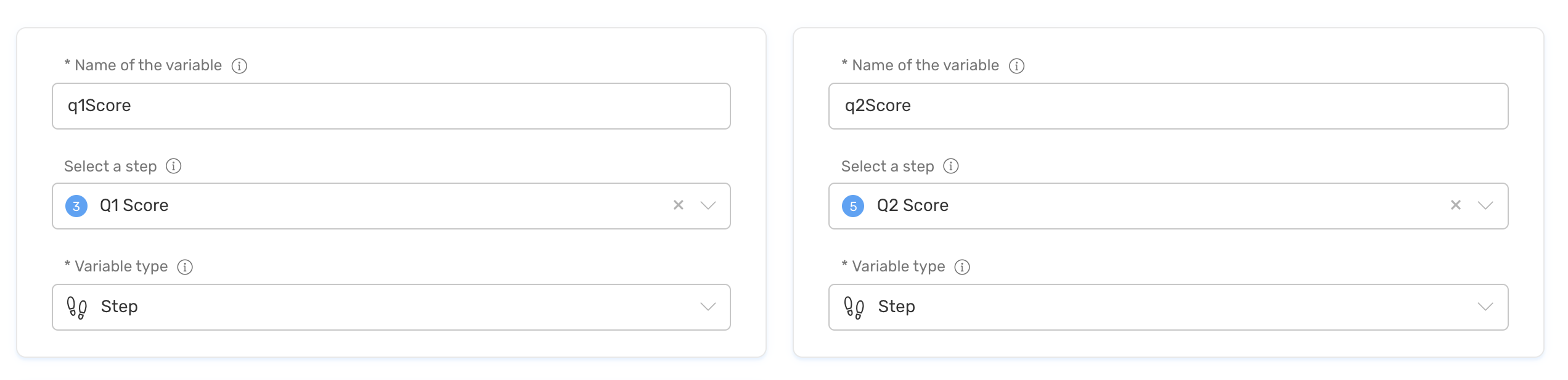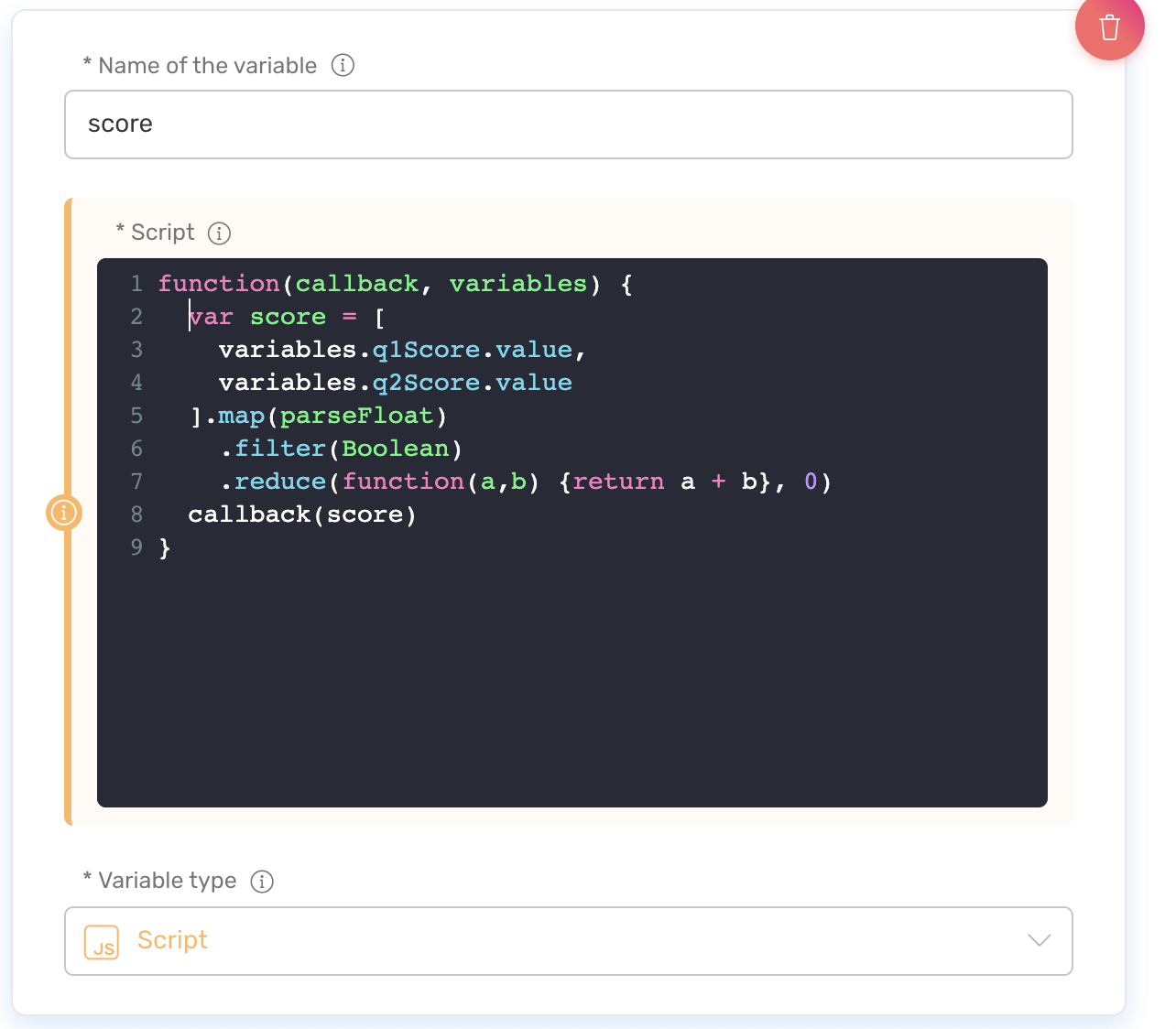It is really easy to build a quiz with Quriobot.

The live demo is located here.

Let's go step by step how to do it.

The basic building block will use the jump logic like this:Each quiz question will need 2 bot steps:

### step 1, for the actual question, using different step types, for example, multiple select or short text:Then it needs 2 responses, one for the correct answer, jumping to the scoring step:another for the incorrect answer, jumping to the next question:### Step 2, and the scoring step, which has to be a Goto step:note the setting Answer value, which would contain the score for this question:Repeat the setup for all the quiz questions.

Next, we need to add bot variables for the score calculations:

add variables of type step q1Score (select Q1 Score step), q2Score (select Q2 Score step), etc for every quiz questionthe last variable will be a bit more involved, as we're going to calculate the overall quiz score, it will be of type script (Javsscript). For that, you'd also need to enable the developer mode (see the header bar:) .`function(callback, variables) {  var score = [    variables.q1Score.value,    variables.q2Score.value  ].map(parseFloat)    .filter(Boolean)    .reduce(function(a,b) {return a + b}, 0)  callback(score)}`

variables.q3Score.value

....

in the list.

In our example, we simply make a sum out of all the scores, but you can have your own custom logic if needed, including the external services, if needed.

The last step would be to display our final score, we use the Exit step type here, which uses our score variable: